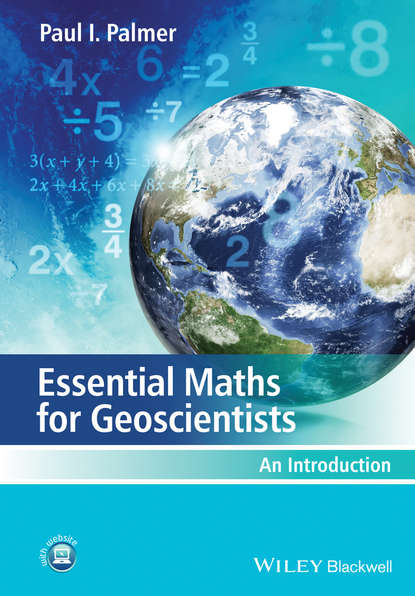Essential Maths for Geoscientists. An Introduction

# Essential Maths for Geoscientists. An Introduction

Автор
Год написания книги
2018
Maths for Geoscientists is an accessible, student-friendly introduction to the essential mathematics required by those students taking degree courses within the Geosciences. Clearly structured throughout, this book carefully guides the student step by step through the mathematics they will encounter and will provide numerous applied examples throughout to enhance students understanding and to place each technique into context. Opening with a chapter explaining the need for studying mathematics within geosciences the book then moves on to cover algebra, equations, solutions, logarithms and exponentials, statistics and probability, trigonometry, vectors and calculus. The final chapter helps to bring it all together and provides the students with sample projects to test their knowledge. Worked applied examples are included in each chapter along with applied problem questions which are a mix of straightforward maths questions, word questions (developing maths to words), and more involved questions that involve the manipulation and interpretation of real and synthetic data.

## Читать онлайн

Авторизуйтесь чтобы можно было оставлять комментарии

## Отзывы

список сообщений пуст